Courses

# Test: Mechanical Properties of Materials - 1

## 10 Questions MCQ Test Topicwise Question Bank for Mechanical Engineering | Test: Mechanical Properties of Materials - 1

Description
This mock test of Test: Mechanical Properties of Materials - 1 for Mechanical Engineering helps you for every Mechanical Engineering entrance exam. This contains 10 Multiple Choice Questions for Mechanical Engineering Test: Mechanical Properties of Materials - 1 (mcq) to study with solutions a complete question bank. The solved questions answers in this Test: Mechanical Properties of Materials - 1 quiz give you a good mix of easy questions and tough questions. Mechanical Engineering students definitely take this Test: Mechanical Properties of Materials - 1 exercise for a better result in the exam. You can find other Test: Mechanical Properties of Materials - 1 extra questions, long questions & short questions for Mechanical Engineering on EduRev as well by searching above.
QUESTION: 1

### Strain is defined as ratio of

Solution:

The strain is defined as the ratio of change in dimension to the original dimension.

If ‘δl’ changes in the length and ‘l’ is the original length, strain = δl/l

Important Terms:

• The ratio of change in length to the original length is called the longitudinal strain.
• The ratio of change in diameter/breadth to original diameter/breadth is called the lateral strain.
• The volumetric strain of a deformed body is defined as the ratio of the change in volume of the body to the deformation to its original volume.
• Shear strain is defined as the strain accompanying a shearing action. It is the angle in radian measure through which the body gets distorted when subjected to external shearing action.
QUESTION: 2

Solution:
QUESTION: 3

### Young's modulus is defined as the ratio of

Solution:

The linear relationship between stress and strain for a bar in simple tension or compression can be expressed by the equation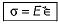in which Eis a constant of proportionality known as modulus of elasticity for the material. The modulus of elasticity is the slope of the stress- strain diagram in the linearly elastic region and its value depends upon the particular material being used.

QUESTION: 4

Match List-I (Material) with List-ll (Young’s modulus):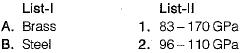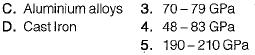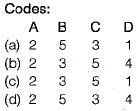Solution: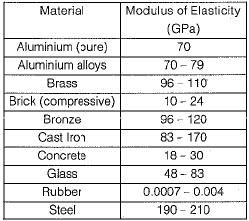QUESTION: 5

The permanent mode of deformation of a material known as ____________

Solution:

Plasticity is defined as the property of a material due to which it is permanently deformed due to loading. Elasticity is the temporary form of deformation. Twinning and Slip are mechanisms of Plastic deformation.

QUESTION: 6

A thin mid steel wire is loaded by adding loads in equal increments till it breaks. The extensions noted with increasing loads will behave as under

Solution:

At first, the strain is proportional to strain or elongation is proportional to the load giving a straight-line relationship.

A further increase in the load after yield load will cause marked deformation in the whole volume of the metal. The maximum load which the specimen can withstand without failure is called the load at the ultimate strength.

QUESTION: 7

Match List-1 (Materia!) with List-ll (Poisson’s Ratio)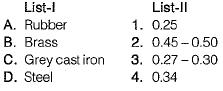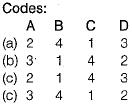Solution:
QUESTION: 8

During a tensile test on a specimen of 1 cm2 cross-section, maximum load observed was 80 kN and area of cross-section at neck was 0.5 cm2. UTS of specimen is

Solution: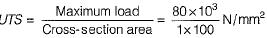= 800 MPa

QUESTION: 9

A prismatic bar of circular cross-section is loaded by tensile forces P= 90 kN. The bar has length L = 3 m and diameter d = 30 mm. If is made of aluminium with modulus of elasticity E = 70 GPa and Poisson’s ratio v = 1/3. What is the increase in volume ΔV of the bar?

Solution: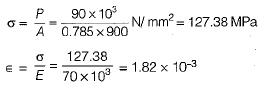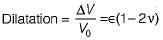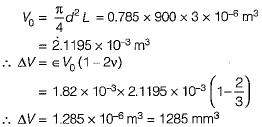QUESTION: 10

Resilience of a material is considered when it is subjected to

Solution:

Resilience represent the ability of the material to absorb energy within the elastic range.